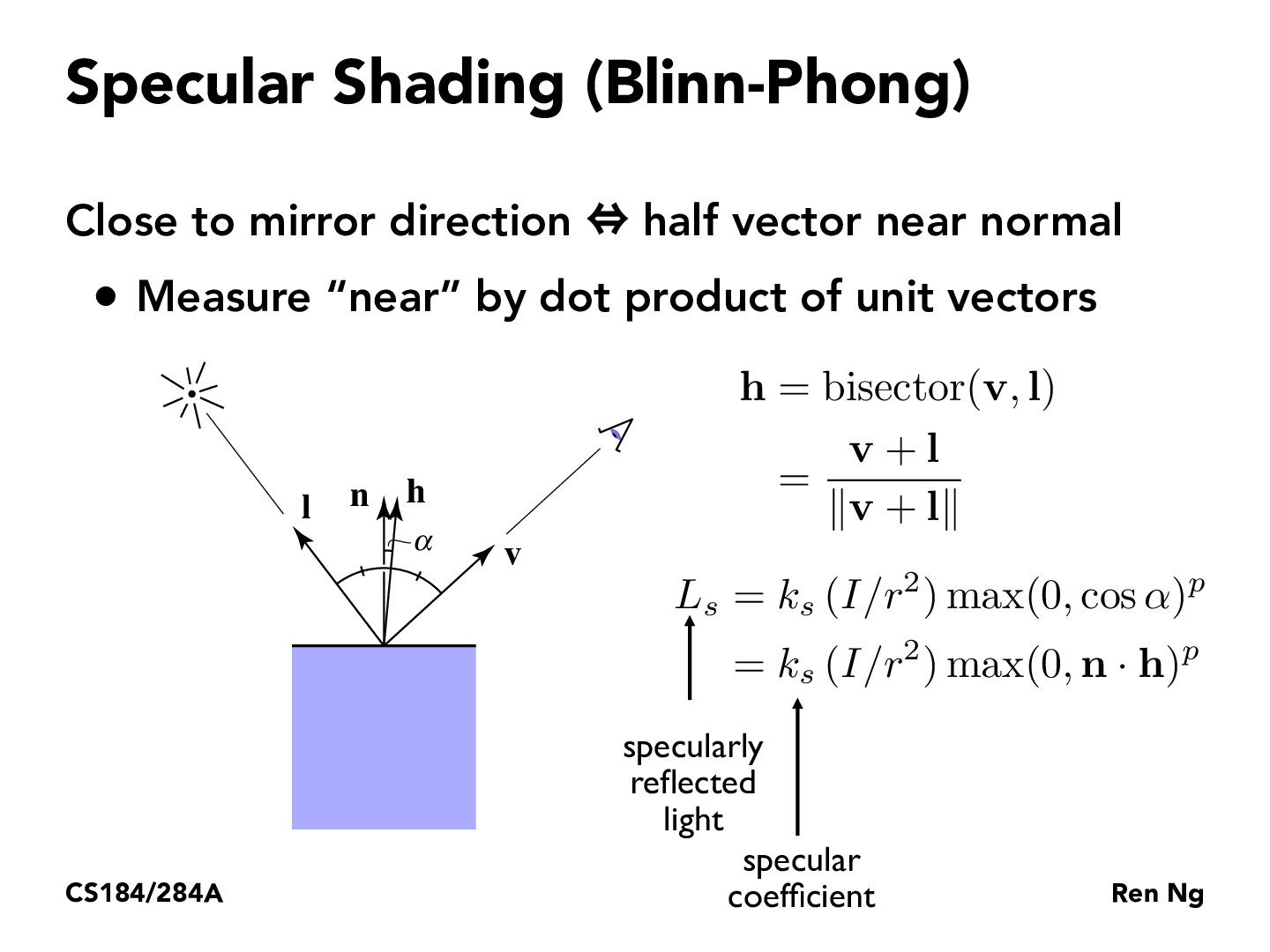You are viewing the course site for a past offering of this course. The current offering may be found here.Lecture 6: Rasterization Pipeline (27)jeffreychen24

I love how the equation works out. If alpha is smaller, then L_s increases. As alpha approaches 180, then L_s would be 0.czhang51

A sort of intuitive way to think about this equation: <n, h> is a measure of how similar vectors n and h are (how small the angle is between them.) And h is in between l and v, so h being near normal means l and v are similar angles away from normal. Which you can imagine is how light reflects off of a surface. I hope this helps make this equation easier to remember for someone.

You must be enrolled in the course to comment